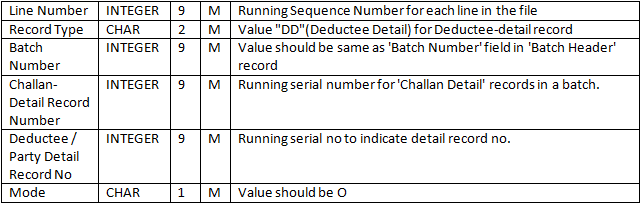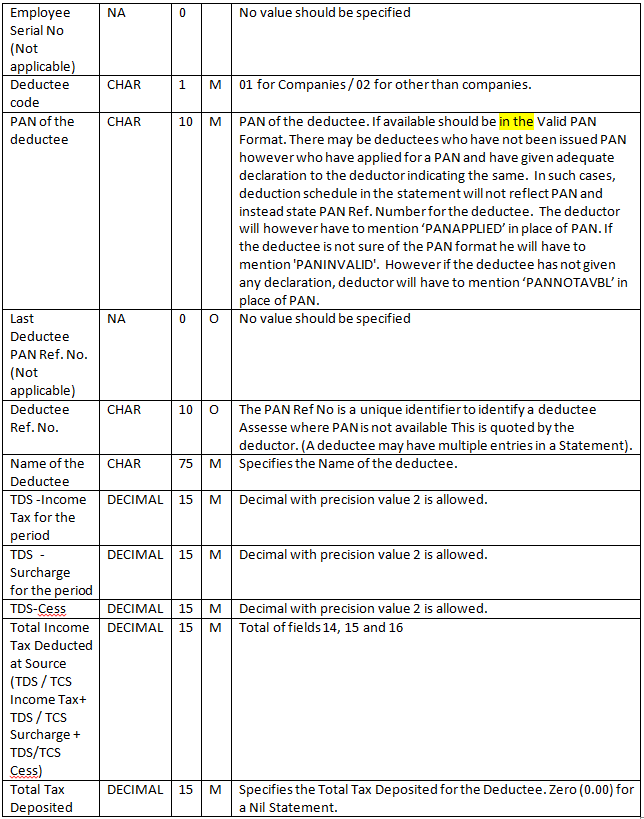# Form 26Q file generated in TDS-Part2

By | October 31, 2014

In our last blog, we discussed about the File header record and Batch header record of form 26Q. In this blog we will discuss on the challan detail and deductee details sections in the form 26Q.
>> Challan details.

Challan details record mainly contain the information remittance done in the TDS module. So it actually stores the information of challan paid for that particular quarter. Please refer the below table which gives you the details of mandatory fields of challan detail sections.

• So the challan details generated in the txt file is in the below format. User can find the challan detail with record type as CD in the starting of the line
3^CD^1^1^1^N^^^^^^62382^^^^0510308^^30092014^^^^26573.00^0.00^0.00^0.00^0.00^26573.00^^26573.00^26573.00^0.00^0.00^26573.00^0.00^0.00^^N^^0.00^200^

>> Deductee details:

Deducted details record mainly contains the information of all the invoices for which TDS is deducted in the particular remittance entry. So below the challan detail line in the TXT one can find detail of all invoices. Please refer the mandatory fields in the deductee details are as follows.• The fields will be display in the text file is as follows.
4^DD^1^1^1^O^^1^^PANNOTAVBL^^^SAP AG^26573.00^0.00^0.00^26573.00^^26573.00^^^265732.00^30092014^30092014^^10.0000^^^^C^^^195^^^^^91^
In this way the FORM 26Q file